26.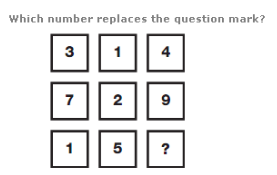A. 6 B. 8 C. 13 D. 4

Explanation: :

On each row, add the values of the left hand and central boxes to give the value in the right hand box.

27.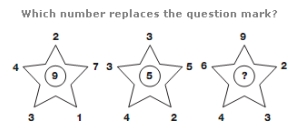A. 11 B. 45 C. 10 D. 21

Explanation: :

In each star diagram, the number in the centre equals the sum of the top three numbers, subtracting the sum of the bottom two numbers.

28.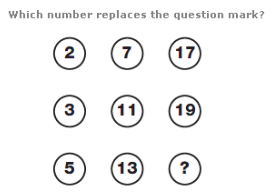A. 20 B. 30 C. 32 D. 23

Explanation: :

Starting top left, and moving down in columns from left to right, the numbers follow the sequence of Prime Numbers from 2 to 23.

29.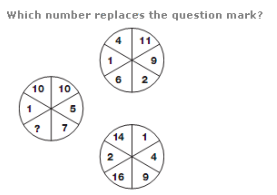A. 10 B. 13 C. 19 D. 21

Explanation: :

Numbers in the segments of the left hand circle equal the difference between numbers in corresponding segments of the two right hand circles.

30.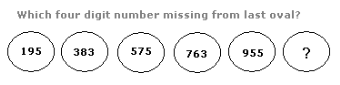A. 1111 B. 2222 C. 4311 D. 1143